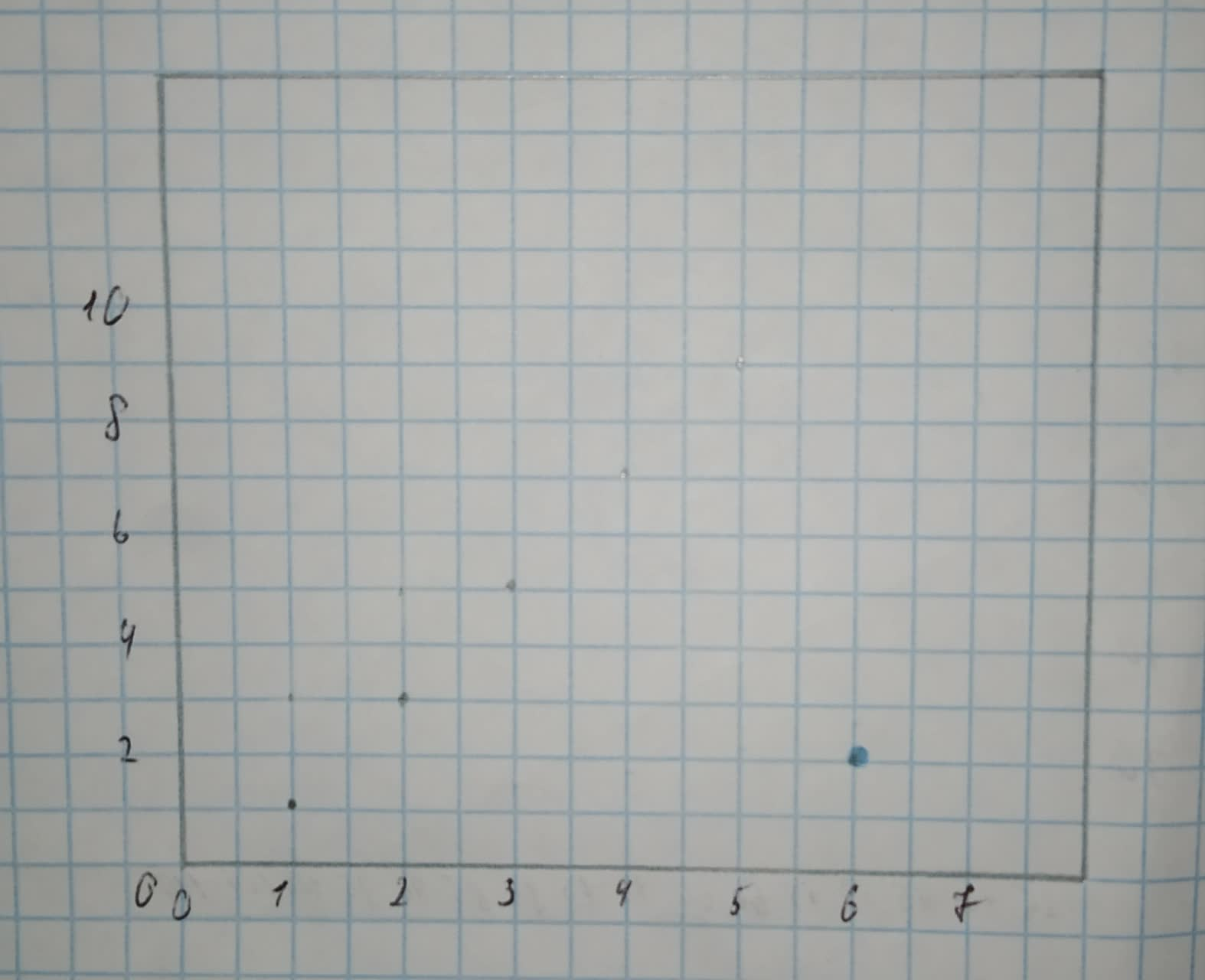# Sketch a scatterplot in which the presence of an outlier decreases the observed correlation between the response and explanatory variables. Indicate on your plot which point is the outlier.he298c 2020-11-05 Answered
Sketch a scatterplot in which the presence of an outlier decreases the observed correlation between the response and explanatory variables. Indicate on your plot which point is the outlier.
You can still ask an expert for help

• Questions are typically answered in as fast as 30 minutes

Solve your problem for the price of one coffee

• Math expert for every subject
• Pay only if we can solve itMalena

Step 1
Let the following data be the initial dataset (without any outliers):
[x 1 2 3 4 5 y 1 3 5 7 9]
Since all points lie on the line $\left[y=2x-1\right]$, the correlation between those two variables is $\left[r=1\right]$ (there is a perfect relationship between the two variables, since there exists a line that passes through all the points, and also, the slope of this line is $\left[{b}_{1}=2>0\right]$ so the association between the two variables is positive which implies that the correlation is also positive).
Let's add the point (6,2) to our initial dataset, so that the data is:
[x 1 2 3 4 5 6 y 1 3 5 7 9 2]
The outlier that we've just added clearly doesn’t belong to the line $\left[y=2x-1\right]$
since $\left[2\ne q2\cdot 6-1=11\right]$.
Therefore, we lost the perfect relationship between the two variables, so the correlation coefficient decreased and the new correlation coefficient is $\left[r<1\right]$.
Here is the scatterplot of the given data (outlier is coloured in blue):